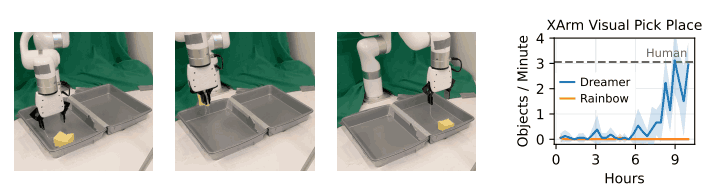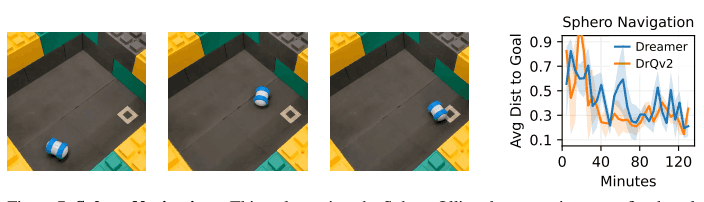# Catch up on the latest AI articles# DayDreamer: Dreamer Is Finally An Actual Robot!

3 main points
✔️ Show that Dreamer can learn on 4 real-world robots
✔️ Enabled a quadruped robot to spin, stand up, and move forward with its back to the ground in about an hour
✔️ The robot could learn to grab an object using an image as input and then place it in a different location using a sparse reward

written by Philipp WuAlejandro EscontrelaDanijar HafnerKen GoldbergPieter Abbeel
(Submitted on 28 Jun 2022)
Comments: Published on arxiv.

Subjects: Robotics (cs.RO); Artificial Intelligence (cs.AI); Machine Learning (cs.LG)code：The images used in this article are from the paper, the introductory slides, or were created based on them.

## first of all

Learning robots to solve complex tasks in the real world has attracted much attention in robotics research in recent years. In particular, deep reinforcement learning (RL) has become possible to improve the robot's behavior and eventually solve complex tasks through repeated trial-and-error. However, learning a robot using Deep RL has the drawbacks that it requires a long period of interaction with the environment and a large number of samples to be collected.

On the other hand, a method called the world model, which has been attracting attention in recent years, learns the environment itself from interaction data with the environment in the past, and can imagine what the result will be if a certain action is taken under a certain situation in that environment. By using this method, for example, planning can be done, and by using a small amount of interaction data with the environment, it is possible to learn the robot's behavior, in other words, to learn policy. The effectiveness of this method has been confirmed especially in games, but its usefulness in the real world has not been demonstrated until now.

In this paper, to confirm this, we applied a world model learning method called Dreamer to the following four robots and showed that they can effectively learn by online learning in the real world. In this article, we explain the Dreamer method and show the results of each robot experiment.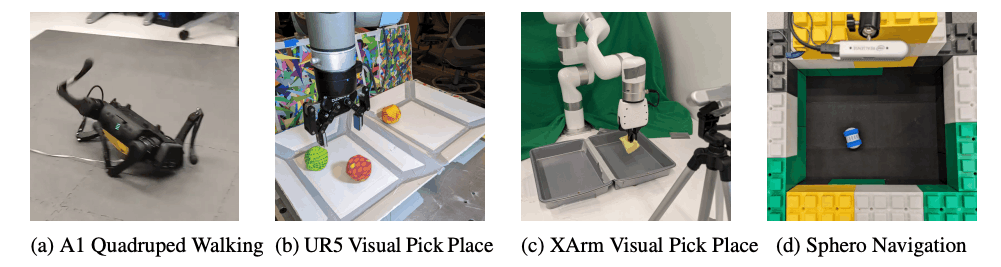## technique

In this paper, we applied Dreamer, a method for learning world models by online learning and learning behaviors at the same time, to a real-world robot. In this chapter, we introduce Dreamer. The following figure shows the overall image of the Dreamer method.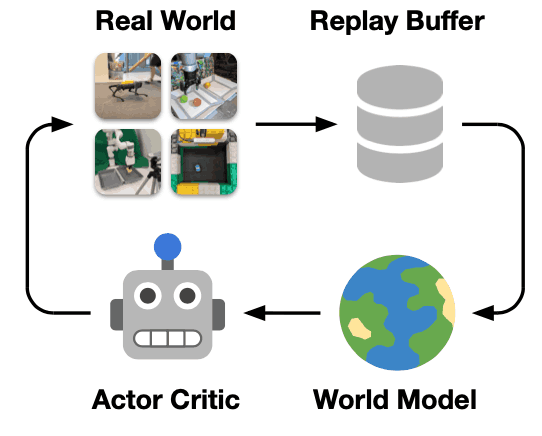Dreamer learns a world model from the experience data of past interactions with the environment and then learns behaviors based on the trajectory predicted from the learned world model using the actor-critic algorithm. Therefore, the behavior itself is not learned from the interaction with the real-world environment, but from the data imagined by the learned world model. In this research, we also separated data collection and model updating, i.e., updating the world model, actor, and critic, and continued to collect data in one thread while updating the model in another thread to make learning more efficient. The model is updated in a separate thread at the same time.

### World Model Learning

First of all, we explain how to train a world model. The following figure shows the overall picture of the world model, which aims to estimate the dynamics of the environment as shown in the figure below. However, if we directly estimate the future image using data such as images, the error between the estimated future image and the actual image tends to be large, which leads to an accumulation of errors when estimating the dynamics of the long-term future. The world model is based on the Recurrent State-Space Model, which consists of the following four networks.

Encoder Network: $enc_{\theta} (s_{t} | s_{t-1}, a_{t-1}, x_{t})$

Decoder Network: $dec_{\theta} (s_{t}) \approx x_{t}$

Dynamics Network: $dyn_{\theta} (s_{t} | s_{t-1}, a_{t-1})$

Reward Network: $rew_{\theta}(s_{t+1}) \approx r_{t}$

The robot is equipped with multiple sensors, for example, the robot's joint angle, force sensor, RGB and depth camera images, etc. The encoder of Dreamer's world model outputs a stochastic representation $Z_{t}$ of these sensors. Therefore, the encoder of the world model of Dreamer outputs the stochastic representation $z_{t}$ by combining this sensor information. Then, the dynamics model outputs the next stochastic representation $z_{t+1}$ using the current state $h_{t}$. The output result is not directly used for learning the behavior. The Reward network is trained to predict these collected rewards.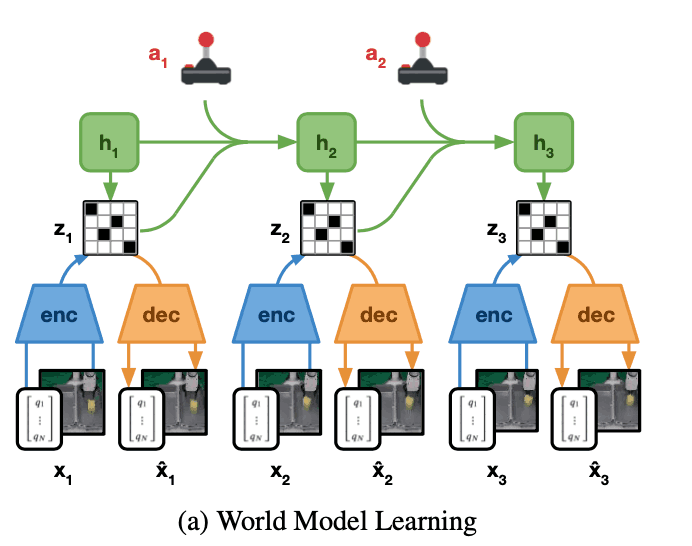### Actor-Critic Learning

While the world model learns a task-independent representation of the dynamics of the environment, the actor-critic algorithm is used to learn task-specific behaviors. The learning uses the rollout estimated in the world model's latent space to learn actions, as the figure below shows. This Actor critic algorithm consists of the following two neural networks.

Actor Network: $\pi (a_{t}| s_{t})$

Critic Network: $v(s_{t})$

Here, the actor-network learns the distribution of actions $a_{t}$ that maximize the reward of the estimated task for each latent space state $s_{t}$. On the other hand, the critic network is trained using temporal difference learning to estimate the total future reward (value) of the task. The learning of the value function by the critic network is important because it takes into account the rewards beyond the planning horizon (H=16). returns as follows ($\lambda$-returns).

$V_{t}^{\lambda} \doteq r_{t} + \gamma((1-\lambda) v(s_{t+1}) + \lambda V_{t+1}^{\lambda}, \quad V_{H}^{lambda} \doteq v(s_{H}))$

Actors are trained with the goal of maximizing value, but also to encourage them to explore the environment during training, thus encouraging them to keep their entropy high. With this in mind, actors are trained using the loss function below.

$\mathcal{L}(\pi)\doteq-\mathrm{E}[\sum_{t=1}^{H} \ln \pi(a_{t} | s_{t}) sg(V_{t}^{\lambda}-v(s_{t})) + \eta \mathrm{H}[\pi(a_{t} | s_{t})]]$

Here, $sg$ indicates that the gradient calculation is stopped. That is, the critic itself is not updated.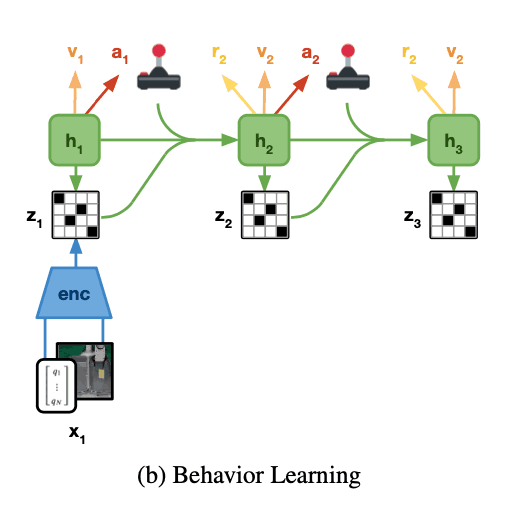## experiment

In this paper, we trained and evaluated Dreamer on four robots. In addition to important tasks such as locomotion, manipulation, and navigation, we evaluated various patterns of these robots' tasks, such as continuous or discontinuous action space, whether the reward is dense or sparse, and whether the proprioceptive information (robot's information, e.g., joint state), images, or other sensors are combined. information (e.g., the robot's information, e.g., joint status), images, and other sensor-associated input.

The purpose of this experiment is to confirm whether the robot's behavior can be obtained more efficiently in the real world by using the world model method. Specifically, we are trying to confirm the following through experiments.

• Whether Dreamer can be applied directly to real robots
• Whether Dreamer is capable of acquiring actions in various robot, sensor modalities, and action space types
• How efficient are Dreamer-based methods compared to other reinforcement learning methods?

### baseline

In the experiments with the A1 quadruped robot, the Soft Actor-Critic (SAC) baseline was used to compare with Dreamer because the action space is continuous and the input is low dimensional information. In our experiments with XArm and UR5 robot, we used DQN as a baseline because the input is images and proprioceptive information, and the action space takes discrete values. In particular, we used a method called Rainbow for training. We also compared UR5 with PPO. Finally, for the Sphero navigation task, we compared the DrQv2 method as a baseline, because images are given as input and the action space is continuous.

### A1 Quadruped Walking

In this experiment, we used a robot called Unitree A1 Robot as shown in the figure below to perform a task in which the robot rotates from a prone position, stands up, and moves forward at a certain speed. In previous papers, we have used domain randomization to learn strategies in the simulation and then transfer them to the real-world robot, a mechanism called a recovery controller that helps the robot avoid dangerous situations, and learning the parameters of an action trajectory generator. In this study, however, we did not use any of these methods. The learning was done using a dense reward function. If you are interested in how the reward function is defined, please refer to equation (5) in the paper.

As a result of one hour of learning, as shown in the figure below, the robot was able to learn a series of movements using Dreamer, such as turning, standing up, and walking forward from a state where the robot was facing away from the ground. In the first 5 minutes, the robot was able to rotate and put its feet on the ground. After 20 minutes, it learned to stand up and finally walk. After an additional 10 minutes of learning, the robot was able to withstand the forces exerted on it by external pushes and was able to get back on its feet quickly after falling. In contrast, SAC was able to turn from a backward position and place his feet on the ground but was unable to stand up and walk.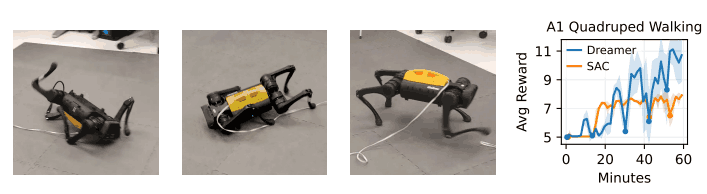### UR5 Multi-Object Visual Pick and Place

The task of grabbing an object and placing it in another bin, as shown below, is important as it is a common task in warehouses. This task is very difficult because it aims to be trained using a sparse reward function, so it must be able to estimate the location of the object from the image and the dynamics of multiple moving objects. The information from the sensors will be the angle of the robot's joints, the position of the gripper and the Cartesian coordinates of the end-effector, and the RGB image. The rewards were a +1 reward when the gripper was detected to be halfway closed, a -1 reward when the object was released into the same bin, and a +10 reward when the object was placed in the opposite bin. The action space consists of the actions of moving the end-effector a certain distance to the X-, Y-, and Z-axis and closing or opening the gripper, which takes discrete values.

Dreamer required 8 hours of learning to be able to grab an average of 2.5 objects per minute. It did not learn very well, especially at the beginning because the reward was sparse, but after 2 hours, its performance started to improve. In contrast, the baseline PPO and Rainbow failed to learn, and although they were able to grasp objects, they quickly let go of them. We believe that these methods require more experience and would be difficult to learn in a real-world setting.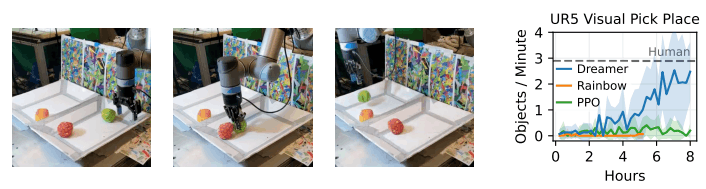### XArm Visual Pick and Place

Similar to the UR5 experiment, the XArm is a relatively inexpensive 7DoF robot that is trained on the task of estimating the location of an object from an image and moving it to another bin. In this experiment, we use a soft object, and the gripper is connected to the object by a string so that the object can be moved, even if it is at the edge of the bin. The reward function is learned using the same sparse reward as in the UR5 experiment. We also used the depth image as input information in addition to the information used in the UR5 experiment.

Dreamer was able to grab an average of 3.1 objects per minute in 10 hours of POLICY and move them to another bin. Also, with Dreamer, when we changed the light conditions, it initially failed to solve the task but was able to quickly adapt after a few hours of learning. In contrast, when using Rainbow, it failed to learn because it required a lot of experience, as in our experiments with UR5.